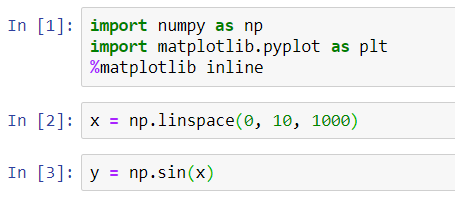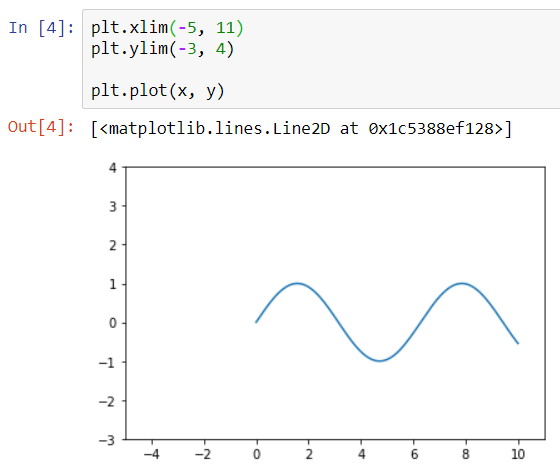When we create a plot in Matplotlib, the axis limits are adjusted automatically by Matplotlib itself without any external interference.

But if we want our own/custom axis dimensions, we have two available functions in Matplotlib, plt.xlim(a,b) and plt.ylim(a,b) for customizing X-axis & Y-axis limits respectively.

Let us begin by importing the Matplotlib library and creating a sinusoid function to be plotted.Let us suppose that we want X-axis ranging from -5 to 11 and Y-axis ranging from -3 to 4.

When we create a plot, we will get something as shown below. We can see that limiting the axis has fixed our figure size.